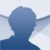Symbolab Android

9.4.0

Symbolab is the math calculator that you have always wanted. It solves any mathematical problem right away. If only everything in life were that simple!

This application’s functioning is extremely easy. Type in the operation and touch the red Go button. And that’s it! You can share a screen capture of the result straight from the app. You can even create graphics; it’s a true wonder. And if you register you will be able to log in to your mathematical accounts whenever you like, and everything will have been saved onto the app.

No problem is too difficult

With Symbolab you will be able to solve all kinds of mathematical problems, whether they be limits, integers, derivatives, equations, functions, trigonometry, and series, as well as matrixes, vectors, ordinary differential equations, algebra, and problems transformed from Laplace. You can insert any problem you want in the bar at the top and also choose from any of the problems suggested by the app.

• Algebra
• Pre-calculus
• Calculus
• Trigonometry
• Functions and graphics
• Matrixes and vectors
• Analytical geometry

This calculator is capable of solving all kinds of mathematical problems. The free version gives you the solution to any problem that you propose, but if you also want to know the steps that the tool has followed to get to the solution, you will have to pay. The paid-for version gives you an ad-free app, unlimited storage, access from any device, and, most interestingly, shows solutions step by step. This is definitely the calculator that we all would have liked to have had back in the days when we were kids at school.

• Performance improvements and bug fixes.